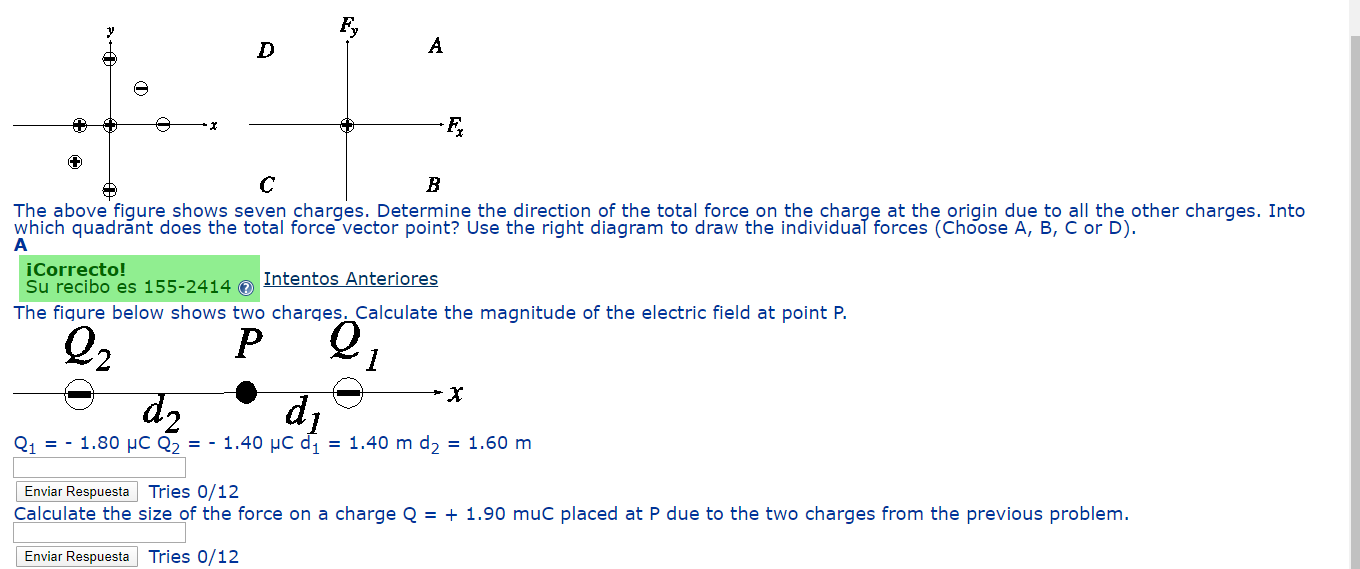Home / Answered Questions / Other / a-the-above-figure-shows-seven-charges-determine-the-direction-of-the-total-force-on-the-charge-at-t-aw660

# (Solved): A) The Above Figure Shows Seven Charges. Determine The Direction Of The Total Force On The Charge At...

A) The above figure shows seven charges. Determine the direction of the total force on the charge at the origin due to all the other charges. Into which quadrant does the total force vector point? Use the right diagram to draw the individual forces (Choose A, B, C or D).

B)The figure below shows two charges. Calculate the magnitude of the electric field at point P.

C)Calculate the size of the force on a charge Q = + 1.90 muC placed at P due to the two charges from the previous problem.The above figure shows seven charges. Determine the direction of the total force on the charge at the origin due to all the other charges. Into which quadrant does the total force vector point? Use the right diagram to draw the individual forces (Choose A, B, C or D). iCorrecto! Su recibo es 155-2414 Intentos Anteriores The figure below shows two charges. Calculate the magnitude of the electric field at point P. Q1 = - 1.80 UC Q2 = - 1.40 uc d = 1.40 m dy = 1.60 m Enviar Respuesta Tries 0/12 Calculate the size of the force on a charge Q = + 1.90 muc placed at P due to the two charges from the previous problem. Enviar Respuesta Tries 0/12

We have an Answer from Expert